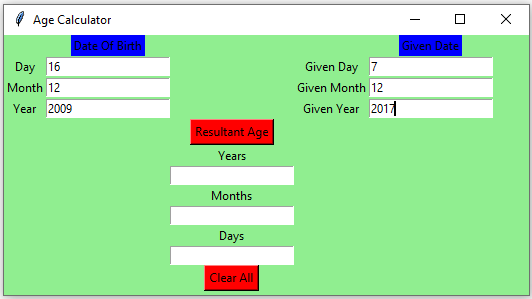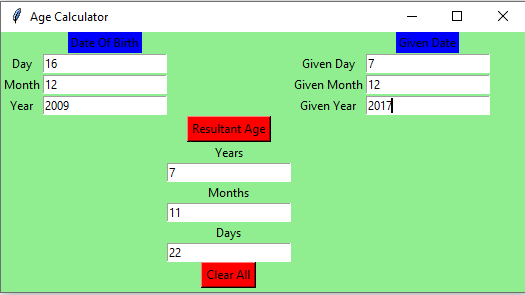# Python: Age Calculator using Tkinter

Prerequisites :Introduction to tkinter

Python offers multiple options for developing a GUI (Graphical User Interface). Out of all the GUI methods, `tkinter` is the most commonly used method. It is a standard Python interface to the Tk GUI toolkit shipped with Python. Python with tkinter outputs the fastest and easiest way to create GUI applications. Now, it’s up to the imagination or necessity of a developer, what he/she wants to develop using this toolkit.

To create a tkinter :

• Importing the module – tkinter
• Create the main window (container)
• Add any number of widgets to the main window.
• Apply the event Trigger on the widgets.

Let’s create a GUI based simple Age Calculator application that can calculate the age with respect to the given date and birth date, given by the user.

Below is the implementation :

 `# import all functions from the tkinter    ` `from` `tkinter ``import` `*` ` `  `# import messagebox class from tkinter ` `from` `tkinter ``import` `messagebox ` ` `  `# Function for clearing the   ` `# contents of all text entry boxes ` `def` `clearAll() : ` ` `  `    ``# deleting the content from the entry box ` `    ``dayField.delete(``0``, END) ` `    ``monthField.delete(``0``, END) ` `    ``yearField.delete(``0``, END) ` `    ``givenDayField.delete(``0``, END) ` `    ``givenMonthField.delete(``0``, END) ` `    ``givenYearField.delete(``0``, END) ` `    ``rsltDayField.delete(``0``, END) ` `    ``rsltMonthField.delete(``0``, END) ` `    ``rsltYearField.delete(``0``, END) ` ` `  `# function for checking error ` `def` `checkError() : ` ` `  `    ``# if any of the entry field is empty ` `    ``# then show an error message and clear  ` `    ``# all the entries ` `    ``if` `(dayField.get() ``=``=` `"``" or monthField.get() == "``" ` `        ``or` `yearField.get() ``=``=` `"``" or givenDayField.get() == "``" ` `        ``or` `givenMonthField.get() ``=``=` `"``" or givenYearField.get() == "``") : ` ` `  `        ``# show the error message ` `        ``messagebox.showerror(``"Input Error"``) ` ` `  `        ``# clearAll function calling ` `        ``clearAll() ` `         `  `        ``return` `-``1` ` `  `# function to calculate Age  ` `def` `calculateAge() : ` ` `  `    ``# check for error ` `    ``value ``=` `checkError() ` ` `  `    ``# if error is occur then return ` `    ``if` `value ``=``=`  `-``1` `: ` `        ``return` `     `  `    ``else` `: ` `         `  `        ``# take a value from the respective entry boxes ` `        ``# get method returns current text as string ` `        ``birth_day ``=` `int``(dayField.get()) ` `        ``birth_month ``=` `int``(monthField.get()) ` `        ``birth_year ``=` `int``(yearField.get()) ` ` `  `        ``given_day ``=` `int``(givenDayField.get()) ` `        ``given_month ``=` `int``(givenMonthField.get()) ` `        ``given_year ``=` `int``(givenYearField.get()) ` `         `  `         `  `        ``# if birth date is greater then given birth_month  ` `        ``# then donot count this month and add 30 to the date so  ` `        ``# as to subtract the date and get the remaining days  ` `        ``month ``=``[``31``, ``28``, ``31``, ``30``, ``31``, ``30``, ``31``, ``31``, ``30``, ``31``, ``30``, ``31``] ` `         `  `        ``if` `(birth_day > given_day): ` `            ``given_month ``=` `given_month ``-` `1` `            ``given_day ``=` `given_day ``+` `month[birth_month``-``1``]  ` `                     `  `                     `  `        ``# if birth month exceeds given month, then  ` `        ``# donot count this year and add 12 to the  ` `        ``# month so that we can subtract and find out  ` `        ``# the difference  ` `        ``if` `(birth_month > given_month): ` `            ``given_year ``=` `given_year ``-` `1` `            ``given_month ``=` `given_month ``+` `12` `                     `  `        ``# calculate day, month, year  ` `        ``calculated_day ``=` `given_day ``-` `birth_day;  ` `        ``calculated_month ``=` `given_month ``-` `birth_month;  ` `        ``calculated_year ``=` `given_year ``-` `birth_year; ` ` `  `        ``# calculated day, month, year write back ` `        ``# to the respective entry boxes ` ` `  `        ``# insert method inserting the   ` `        ``# value in the text entry box. ` `         `  `        ``rsltDayField.insert(``10``, ``str``(calculated_day)) ` `        ``rsltMonthField.insert(``10``, ``str``(calculated_month)) ` `        ``rsltYearField.insert(``10``, ``str``(calculated_year)) ` `     `  ` `  `# Driver Code ` `if` `__name__ ``=``=` `"__main__"` `: ` ` `  `    ``# Create a GUI window ` `    ``gui ``=` `Tk() ` ` `  `    ``# Set the background colour of GUI window   ` `    ``gui.configure(background ``=` `"light green"``) ` ` `  `    ``# set the name of tkinter GUI window  ` `    ``gui.title(``"Age Calculator"``) ` ` `  `     ``# Set the configuration of GUI window ` `    ``gui.geometry(``"525x260"``) ` ` `  `    ``# Create a Date Of Birth : label  ` `    ``dob ``=` `Label(gui, text ``=` `"Date Of Birth"``, bg ``=` `"blue"``) ` ` `  `    ``# Create a Given Date : label ` `    ``givenDate ``=` `Label(gui, text ``=` `"Given Date"``, bg ``=` `"blue"``) ` ` `  `    ``# Create a Day : label ` `    ``day ``=` `Label(gui, text ``=` `"Day"``, bg ``=` `"light green"``) ` ` `  `    ``# Create a Month : label ` `    ``month ``=` `Label(gui, text ``=` `"Month"``, bg ``=` `"light green"``) ` ` `  `    ``# Create a Year : label ` `    ``year ``=` `Label(gui, text ``=` `"Year"``, bg ``=` `"light green"``) ` ` `  `    ``# Create a Given Day : label ` `    ``givenDay ``=` `Label(gui, text ``=` `"Given Day"``, bg ``=` `"light green"``) ` ` `  `    ``# Create a Given Month : label ` `    ``givenMonth ``=` `Label(gui, text ``=` `"Given Month"``, bg ``=` `"light green"``) ` ` `  `    ``# Create a Given Year : label ` `    ``givenYear ``=` `Label(gui, text ``=` `"Given Year"``, bg ``=` `"light green"``) ` ` `  `    ``# Create a Years : label ` `    ``rsltYear ``=` `Label(gui, text ``=` `"Years"``, bg ``=` `"light green"``) ` ` `  `    ``# Create a Months : label ` `    ``rsltMonth ``=` `Label(gui, text ``=` `"Months"``, bg ``=` `"light green"``) ` ` `  `    ``# Create a Days : label ` `    ``rsltDay ``=` `Label(gui, text ``=` `"Days"``, bg ``=` `"light green"``) ` ` `  `    ``# Create a Resultant Age Button and attached to calculateAge function ` `    ``resultantAge ``=` `Button(gui, text ``=` `"Resultant Age"``, fg ``=` `"Black"``, bg ``=` `"Red"``, command ``=` `calculateAge) ` ` `  `    ``# Create a Clear All Button and attached to clearAll function ` `    ``clearAllEntry ``=` `Button(gui, text ``=` `"Clear All"``, fg ``=` `"Black"``, bg ``=` `"Red"``, command ``=` `clearAll) ` ` `  `    ``# Create a text entry box for filling or typing the information.   ` `    ``dayField ``=` `Entry(gui) ` `    ``monthField ``=` `Entry(gui) ` `    ``yearField ``=` `Entry(gui) ` `     `  `    ``givenDayField ``=` `Entry(gui) ` `    ``givenMonthField ``=` `Entry(gui) ` `    ``givenYearField ``=` `Entry(gui) ` `     `  `    ``rsltYearField ``=` `Entry(gui) ` `    ``rsltMonthField ``=` `Entry(gui) ` `    ``rsltDayField ``=` `Entry(gui) ` ` `  ` `  `    ``# grid method is used for placing   ` `    ``# the widgets at respective positions   ` `    ``# in table like structure . ` `    ``dob.grid(row ``=` `0``, column ``=` `1``) ` `     `  `    ``day.grid(row ``=` `1``, column ``=` `0``) ` `    ``dayField.grid(row ``=` `1``, column ``=` `1``) ` `     `  `    ``month.grid(row ``=` `2``, column ``=` `0``) ` `    ``monthField.grid(row ``=` `2``, column ``=` `1``) ` `     `  `    ``year.grid(row ``=` `3``, column ``=` `0``) ` `    ``yearField.grid(row ``=` `3``, column ``=` `1``) ` `     `  `    ``givenDate.grid(row ``=` `0``, column ``=` `4``) ` `     `  `    ``givenDay.grid(row ``=` `1``, column ``=` `3``) ` `    ``givenDayField.grid(row ``=` `1``, column ``=` `4``) ` `     `  `    ``givenMonth.grid(row ``=` `2``, column ``=` `3``) ` `    ``givenMonthField.grid(row ``=` `2``, column ``=` `4``) ` `     `  `    ``givenYear.grid(row ``=` `3``, column ``=` `3``) ` `    ``givenYearField.grid(row ``=` `3``, column ``=` `4``) ` `     `  `    ``resultantAge.grid(row ``=` `4``, column ``=` `2``) ` `     `  `    ``rsltYear.grid(row ``=` `5``, column ``=` `2``) ` `    ``rsltYearField.grid(row ``=` `6``, column ``=` `2``) ` `     `  `    ``rsltMonth.grid(row ``=` `7``, column ``=` `2``) ` `    ``rsltMonthField.grid(row ``=` `8``, column ``=` `2``) ` `     `  `    ``rsltDay.grid(row ``=` `9``, column ``=` `2``) ` `    ``rsltDayField.grid(row ``=` `10``, column ``=` `2``) ` ` `  `    ``clearAllEntry.grid(row ``=` `12``, column ``=` `2``) ` ` `  `    ``# Start the GUI ` `    ``gui.mainloop()     `

Output :My Personal Notes arrow_drop_upCheck out this Author's contributed articles.

If you like GeeksforGeeks and would like to contribute, you can also write an article using contribute.geeksforgeeks.org or mail your article to contribute@geeksforgeeks.org. See your article appearing on the GeeksforGeeks main page and help other Geeks.

Please Improve this article if you find anything incorrect by clicking on the "Improve Article" button below.